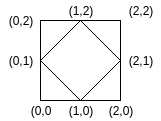# Count the number of rhombi possible inside a rectangle of given size

Given a rectangle of height H and width W which has the bottom left corner at (0, 0). The task is to count the number of distinct Rhombi that have all points inside or on the border of the rectangle satisfying the following conditions exists:

• Have non-zero area.
• Have diagonals parallel to the x and y axes.
• Have integer coordinates.

Examples:

Input: H = 2, W = 2
Output: 2
There is only one rhombus possible with coordinates (0, 1), (1, 0), (2, 1) and (1, 2).Input: H = 4, W = 4
Output: 16

## Recommended: Please try your approach on {IDE} first, before moving on to the solution.

Approach: Since the diagonals are parallel to the axis, let’s try fixing the diagonals and creating rhombi on them. For the rhombus to have integer coordinates, the length of the diagonals must be even. Let’s fix the length of the diagonals to i and j, the number of rhombi we can form with these diagonal lengths inside the rectangle would be (H – i + 1) * (W – j + 1). Thus, we iterate over all possible values of i and j and update the count.

Below is the implementation of the above approach:

## C++

 `// C++ implementation of the approach ` `#include ` `using` `namespace` `std; ` ` `  `// Function to return the count of rhombi possible ` `long` `long` `countRhombi(``int` `h, ``int` `w) ` `{ ` `    ``long` `long` `ct = 0; ` ` `  `    ``// All possible diagonal lengths ` `    ``for` `(``int` `i = 2; i <= h; i += 2) ` `        ``for` `(``int` `j = 2; j <= w; j += 2) ` ` `  `            ``// Update rhombi possible with ` `            ``// the current diagonal lengths ` `            ``ct += (h - i + 1) * (w - j + 1); ` ` `  `    ``// Return the total count ` `    ``// of rhombi possible ` `    ``return` `ct; ` `} ` ` `  `// Driver code ` `int` `main() ` `{ ` `    ``int` `h = 2, w = 2; ` ` `  `    ``cout << countRhombi(h, w); ` ` `  `    ``return` `0; ` `} `

## Java

 `// Java implementation of the approach ` `import` `java.io.*; ` ` `  `class` `GFG  ` `{ ` `     `  `// Function to return the count of rhombi possible ` `static` `int` `countRhombi(``int` `h, ``int` `w) ` `{ ` `    ``int` `ct = ``0``; ` ` `  `    ``// All possible diagonal lengths ` `    ``for` `(``int` `i = ``2``; i <= h; i += ``2``) ` `        ``for` `(``int` `j = ``2``; j <= w; j += ``2``) ` ` `  `            ``// Update rhombi possible with ` `            ``// the current diagonal lengths ` `            ``ct += (h - i + ``1``) * (w - j + ``1``); ` ` `  `    ``// Return the total count ` `    ``// of rhombi possible ` `    ``return` `ct; ` `} ` ` `  `    ``// Driver code ` `    ``public` `static` `void` `main (String[] args)  ` `    ``{ ` `    ``int` `h = ``2``, w = ``2``; ` `    ``System.out.println (countRhombi(h, w)); ` `    ``} ` `} ` ` `  `// This code is contributed by jit_t `

## Python 3

 `# Python 3 implementation of the approach ` ` `  `# Function to return the count of  ` `# rhombi possible ` `def` `countRhombi(h, w): ` ` `  `    ``ct ``=` `0``; ` ` `  `    ``# All possible diagonal lengths ` `    ``for` `i ``in` `range``(``2``, h ``+` `1``, ``2``): ` `        ``for` `j ``in` `range``(``2``, w ``+` `1``, ``2``): ` ` `  `            ``# Update rhombi possible with ` `            ``# the current diagonal lengths ` `            ``ct ``+``=` `(h ``-` `i ``+` `1``) ``*` `(w ``-` `j ``+` `1``) ` ` `  `    ``# Return the total count ` `    ``# of rhombi possible ` `    ``return` `ct ` ` `  `# Driver code ` `if` `__name__ ``=``=` `"__main__"``: ` ` `  `    ``h ``=` `2` `    ``w ``=` `2` ` `  `    ``print``(countRhombi(h, w)) ` ` `  `# This code is contributed by ita_c `

## C#

 `// C# program to find the frequency of  ` `// minimum element in the array  ` `using` `System; ` ` `  `class` `GFG  ` `{  ` `         `  `    ``// Function to return the count ` `    ``// of rhombi possible  ` `    ``static` `int` `countRhombi(``int` `h, ``int` `w)  ` `    ``{  ` `        ``int` `ct = 0;  ` `     `  `        ``// All possible diagonal lengths  ` `        ``for` `(``int` `i = 2; i <= h; i += 2)  ` `            ``for` `(``int` `j = 2; j <= w; j += 2)  ` `     `  `                ``// Update rhombi possible with  ` `                ``// the current diagonal lengths  ` `                ``ct += (h - i + 1) * (w - j + 1);  ` `     `  `        ``// Return the total count  ` `        ``// of rhombi possible  ` `        ``return` `ct;  ` `    ``}  ` `     `  `    ``// Driver code  ` `    ``public` `static` `void` `Main()  ` `    ``{  ` `        ``int` `h = 2, w = 2;  ` `         `  `        ``Console.WriteLine(countRhombi(h, w));  ` `    ``}  ` `}  ` ` `  `// This code is contributed by Ryuga  `

## PHP

 ` `

Output:

```1
```

Time Complexity: O(H * W)

Attention reader! Don’t stop learning now. Get hold of all the important DSA concepts with the DSA Self Paced Course at a student-friendly price and become industry ready.

My Personal Notes arrow_drop_upCheck out this Author's contributed articles.

If you like GeeksforGeeks and would like to contribute, you can also write an article using contribute.geeksforgeeks.org or mail your article to contribute@geeksforgeeks.org. See your article appearing on the GeeksforGeeks main page and help other Geeks.

Please Improve this article if you find anything incorrect by clicking on the "Improve Article" button below.